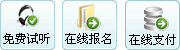﻿ 乘法密码-信息安全工程师教材 - 综合知识 - 信管网

(2) 乘法密码

f(αi)=bi=aj

j=ik  mod  n                             (2-4)

f(A)=f(C)=f(E)=f{G)=f(K)=f{M)=f{O)=f{Q)=f{S)=f{U)=f{W)=f{Y)=A

f(B)=f(D)=f(H)=f(J)=f(L)=f(N)=f(P)=f(R)=f(T)=f(V)=f(X)=f(Z)=N

B={A,N,A,N,A,N,A,N,A,N,A,N,A,N,A,N,A,N,A,N,A,N,A,N,A,N,}

A={A，B，C，D，E，F，G，H，I，J，K，L，M，N，O，P，Q，R， S，T，U，V，W，X，Y，Z}

B={A，F，K，P，U，Z，E，J，O，T，Y，D，I，N，S，X，C，H，M，R， W，B，G，L，Q，V}

 发表评论  查看完整评论

### 相关内容8年专注信管，信管教育领跑者，信管网优势信息安全工程师培训（自有资料+串讲+系统课程）免费试听信管网信息系统项目管理师课程全国前50名高分学员访谈：董丽(174)、李思...信息系统项目管理师培训课程(通关班)信管、集成、系规、安全，专业成就梦想信息安全工程师历年真题与答案汇总中级系统集成项目管理工程师培训课程系统规划与管理师培训课程（自有资料+直播）软题库：软考在线题库、支持手机答题